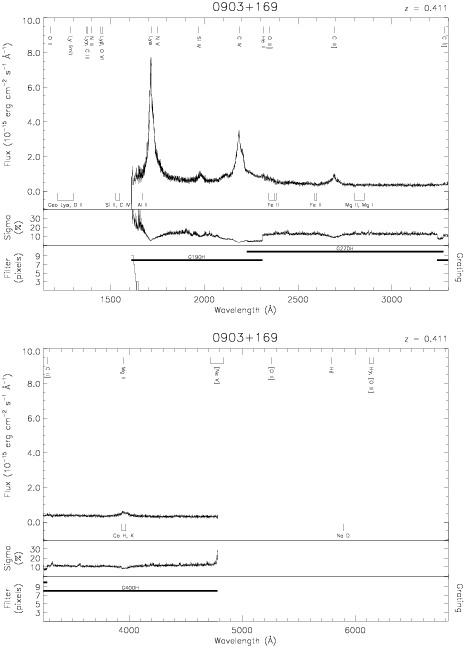Download dataset
TELESCOP= 'HST     '
INSTRUME= 'FOS     '
EQUINOX =               2000.0
DATE-OBS= '1992-05-01T03:36:40'
RA_PNT  =       136.6327500000
DEC_PNT =        16.7700555556
PA_PNT  =       205.0110000000
OBJECT  = '0903+169'
ROOTNM01= 'Y0PE0602T'
GRNDMD01= 'SPECTROSCOPY'
DETECT01= 'AMBER   '
APERID01= 'A-1     '
FGWAID01= 'H19     '
ROOTNM02= 'Y0PE0603T'
GRNDMD02= 'SPECTROSCOPY'
DETECT02= 'AMBER   '
APERID02= 'A-1     '
FGWAID02= 'H19     '
ROOTNM03= 'Y0PE0604T'
GRNDMD03= 'SPECTROSCOPY'
DETECT03= 'AMBER   '
APERID03= 'A-1     '
FGWAID03= 'H19     '
ROOTNM04= 'Y0PE0605T'
GRNDMD04= 'SPECTROSCOPY'
DETECT04= 'AMBER   '
APERID04= 'A-1     '
FGWAID04= 'H27     '
ROOTNM05= 'Y0PE0606T'
GRNDMD05= 'SPECTROSCOPY'
DETECT05= 'AMBER   '
APERID05= 'A-1     '
FGWAID05= 'H27     '
ROOTNM06= 'Y0PE0607T'
GRNDMD06= 'SPECTROSCOPY'
DETECT06= 'AMBER   '
APERID06= 'A-1     '
FGWAID06= 'H40     '